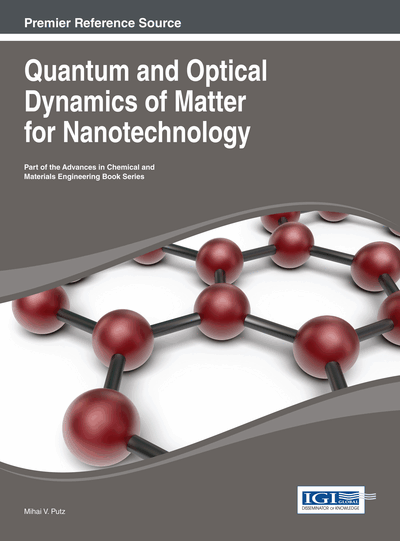# General Concepts and Theories in X-Ray Diffraction

DOI: 10.4018/978-1-4666-4687-2.ch011

## Abstract

The various forms of the X-Ray Diffraction (XRD) theory in the crystal are considered as perfect or imperfect for the version “perfect on portions” (i.e. with slight deformations or with a constant deformation gradient), following historical developments and performances of the models.
Chapter Preview
Top

## 11.2 Geometric Vs. Dynamic Theories

The feature of the dynamic approach is that in 1912 Laue has seen the diffracted amplitude through a three-dimensional network infinite periodic as a simple sum of the diffracted wave amplitudes to each atom of the network in part (a Fourier transform, the analogous of the optical diffraction) neglecting the secondary interaction of the waves in the diffraction process with the crystal.

Considering the electronic density distribution for such an environment,, and the electronic density in a cell, the connection between them is given by the convolution through the Dirac distribution located in the nodes of the direct network such as:

(11.1) the total distribution being limited by the physical shape of the crystal, by:

(11.2)

According to this approach, the total scattering amplitude will be written as a simple continuous sum of the amplitudes scattered by each diffraction center considered, in the reciprocal space:

(11.3) which is nothing else but the Fourier transform of the expression (11.2) through the whole diffraction space selecting only the diffraction vectors which are found on the reflection sphere.

Replacing (11.1) and (11.2) in (11.3) and using the properties of the Fourier transform, for the total scattering amplitude through the medium is obtained (Authier & Malgrange 1998):

(11.4)

This result has some consequences characteristic to the geometrical approach of the X-ray diffraction (Authier & Malgrange 1998; Authier 1960; Ewald 1965, 1969):

## Complete Chapter List

Search this Book:
Reset